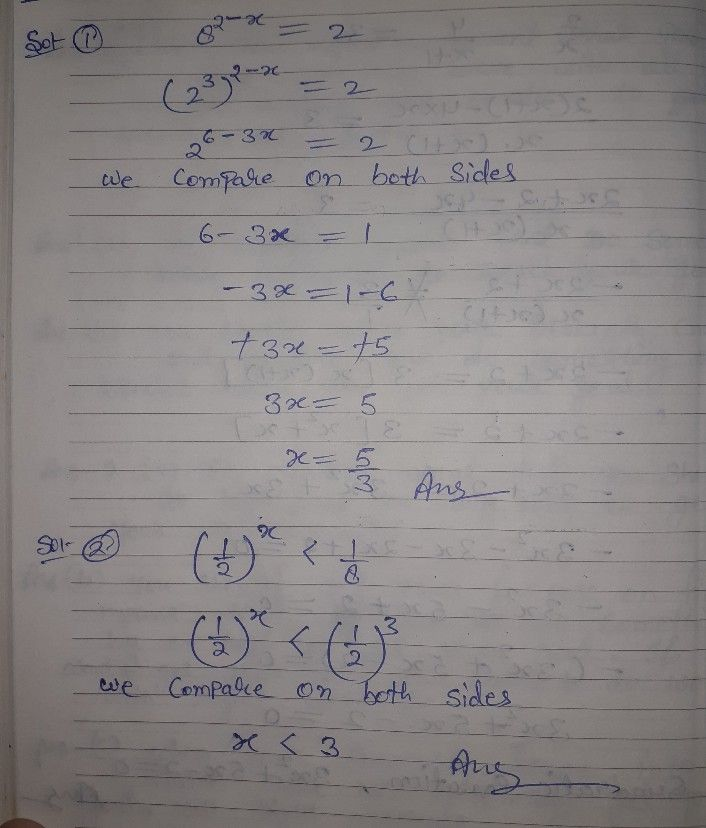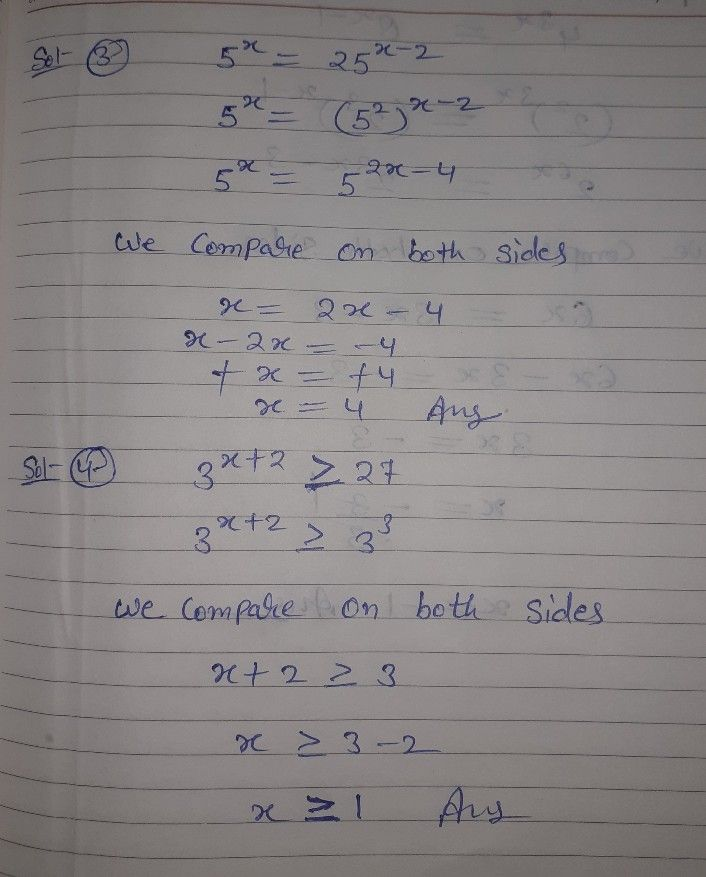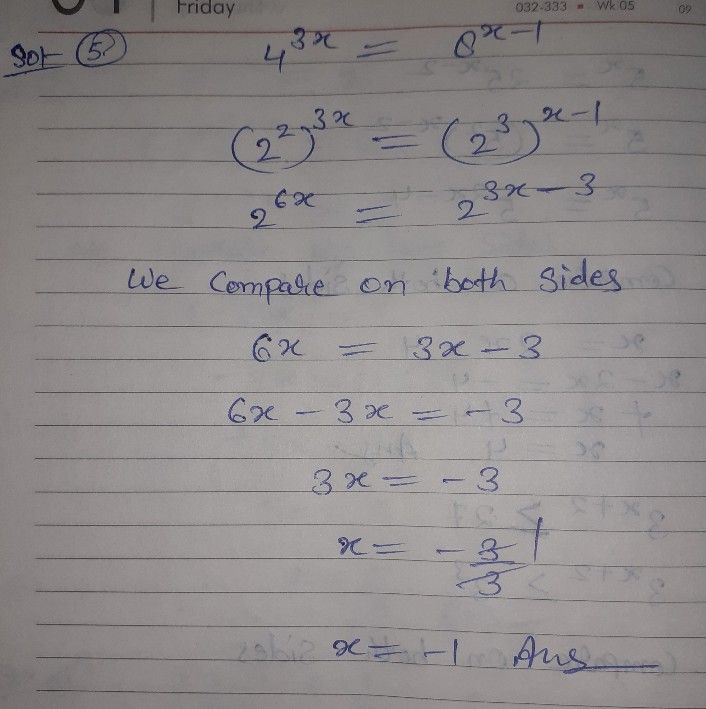Symbol
ProblemActivity $18.2$ Find the Missing x! Solve for the values of $X$ for each of the following exponential equations and inequalities. $1.$ $8^{2-x}=2$ $2.$ $\left(\dfrac {1} {2}\right)^{x}<\dfrac {1} {8}$ $3$ $5^{x}.=25^{x-2}$ $4$ $3^{x+2}\geq 27$ $5$ $4^{3x}=8^{x-1}$